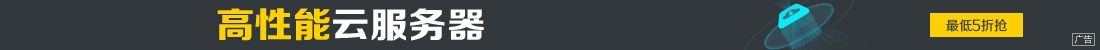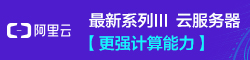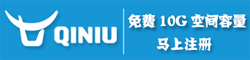# vbscript include的办法实现代码第1/2页

••Set WSHShell = CreateObject("WScript.Shell")
WSHShell.Run "wscript c:\Test.vbs param1", , True

object.Run(strCommand, [intWindowStyle], [bWaitOnReturn])

Set oArgs = WScript.Arguments
For i = 0 to oArgs.Count - 1
WScript.Echo oArgs(i)
Next

' searches for and returns the value of a command line argument of the form
' /argName:value from the supplied array. erases the entry in the array so
' that only untouched entries remain.

function GetArgValue(argName, args())
dim a
dim v
dim argNameLength
dim x
dim argCount
dim fullArgName

fullArgName = "/" & argName & ":"
argCount = Ubound(args)

' Get the length of the argname we are looking for
argNameLength = Len(fullArgName)
GetArgValue = "" ' default to nothing

for x = 0 To argCount
if Len(args(x)) >= argNameLength then

a = Mid(args(x), 1, argNameLength)
if UCase(a) = UCase(fullArgName) then

' erase it so we can look for unknown args later
v = args(x)
args(x) = ""

if Len(v) > argNameLength then
GetArgValue = Mid(v, argNameLength + 1)
exit function
else
GetArgValue = ""
exit function
end if
end if
end if
next
end function

Sub Include(sInstFile)
Dim oFSO, f, s
Set oFSO = CreateObject("Scripting.FileSystemObject")
Set f = oFSO.OpenTextFile(sInstFile)
f.Close
ExecuteGlobal s
End Sub

Sub Include(sInstFile)
Dim oFSO, f, s
Set oFSO = CreateObject("Scripting.FileSystemObject")
Set f = oFSO.OpenTextFile(sInstFile)
f.Close
ExecuteGlobal s
End Sub